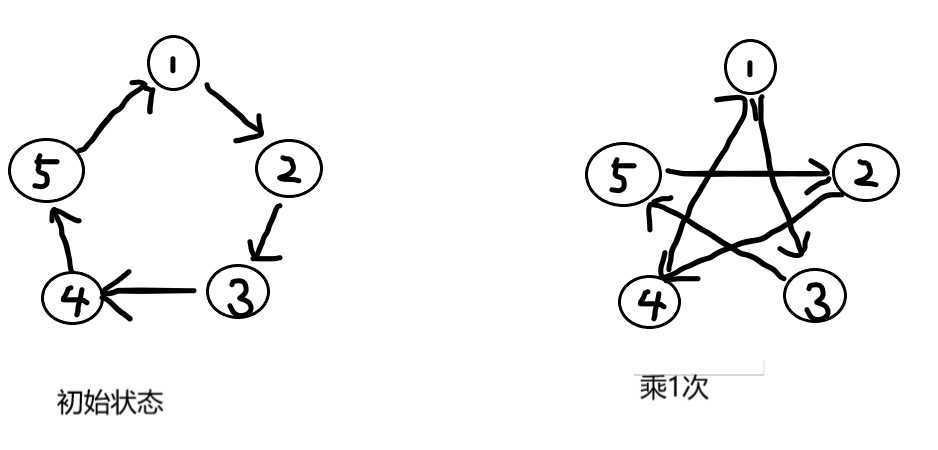# CF1327D Infinite Path``````#include <iostream>
#include <cstdio>
#include <cstring>
#include <algorithm>
#include <cmath>
#include <queue>
using namespace std;

typedef long long ll;

const int inf=0x3f3f3f3f;
const ll llinf=0x3f3f3f3f3f3f3f3f;
const double pi=acos(-1);
const int mod=998244353;

int nxt,col;
int vis;
int que;

int func(int top)
{
for(int i=1;i<=top;i++) if(top%i==0)
{
for(int j=0;j<i;j++)
{
int flag=que[j];
for(int k=j;k<top;k+=i)
if(flag!=que[k]) flag=0;
if(flag) return i;
}
}
return -1;
}

int main()
{
int T;
scanf("%d",&T);
while(T--)
{
int n;
scanf("%d",&n);
for(int i=0;i<n;i++) scanf("%d",&nxt[i]);
for(int i=0;i<n;i++) scanf("%d",&col[i]);
for(int i=0;i<n;i++) vis[i]=0;
int ans=inf;
for(int i=0;i<n;i++) if(!vis[i])
{
int t=i,top=0;
while(!vis[t])
{
que[top++]=col[t];
vis[t]=1;
t=nxt[t]-1;
}
ans=min(ans,func(top));
}
printf("%d\n",ans);
}
return 0;
}

``````

CF1327D Infinite Path

(0)
(0)